# 0. Note

The source code of the Windows API was not examined, so it is an experimental verification.
The code example is C #.

For those who say “code is good”, to the last chapter.
Behavior on multiple monitors has not been verified.

# 1. Moving the mouse cursor using SendInput with absolute coordinates

If you want to move the mouse cursor to a specified coordinate on the desktop regardless of the current mouse cursor position, specify MOUSEEVENTF_ABSOLUTE and use SendInput (Windows API) to control the mouse.

• If you just want to move it, you can set the coordinates in Cursor.Position. If you want to use the mouse button operation together, you should use SendInput.

The streets are full of codes that perform the following conversions, but if you use them, they will deviate from the originally intended coordinates (x, y) due to rounding errors.
(Examples 2 and 3 are usually not a concern because I think the deviation will be 1 pixel, but it can be a problem depending on the scene.)

#### NG example 1



absX = x * (65535 / Screen.PrimaryScreen.Bounds.Width);
absY = y * (65535 / Screen.PrimaryScreen.Bounds.Height);


For NG example 1, since / is an integer division in C language and C #, it depends on the Width and Height of the monitor when the coefficient (65535 / Screen.PrimaryScreen.Bounds.Width) is calculated. It will have an error. [^ 1]

If you are familiar with it, use NG example 2.

#### NG example 2



absX = (x * 65535) / Screen.PrimaryScreen.Bounds.Width;
absY = (y * 65535) / Screen.PrimaryScreen.Bounds.Height;


Or, since the numerator is subtracting 1 (from 65536), I think there are people who also subtract 1 in the denominator and use the following.

#### NG example 3



absX = (x * 65535) / (Screen.PrimaryScreen.Bounds.Width - 1);
absY = (y * 65535) / (Screen.PrimaryScreen.Bounds.Height - 1);


# 2. The specification description of Send Input on the official website is not cool in the first place

See NG example 2 and NG example 3, and write in “No, Microsoft official website I’m sure there are people who think, “Isn’t it right?” (I thought so too.)

The description of Official site is excerpted.

If MOUSEEVENTF_ABSOLUTE value is specified,
dx and dy contain normalized absolute coordinates between 0 and 65,535.
The event procedure maps these coordinates onto the display surface.
Coordinate (0,0) maps onto the upper-left corner of the display surface;
coordinate (65535,65535) maps onto the lower-right corner.
In a multimonitor system, the coordinates map to the primary monitor.

Looking at this, it is as if (65535,65535) and (Width, Height) or (Width-1, Height-1) of the primary monitor. > Seems to correspond.

However, when I actually try it with the code of NG example 2 and NG example 3, it shifts.
The experiment of the case of deviation is omitted in this article, but it can be confirmed by using the code of this article .

# 3. I examined the relationship between the specified coordinates and the coordinates after the actual movement.

In Chapter 3, we will examine where the mouse cursor coordinates will be after executing SendInput for the specified coordinates.
In this chapter, we will get an expression to get the coordinate system in pixels from the coordinate system (0,0)-(65535 (?), 65535 (?)) of the world of SendInput.

The final result is a transformation in the opposite direction. We’ll cover that in Chapter 4.

## 3.1. Conclusion

As a result of verification by experiment, (0,0)-(6553 6 , 6553 6 ) corresponds to (0,0)-(Width, Height) of the primary monitor. There is a relationship. The rounding direction is rounded down.
In other words, the SendInput API seems to internally perform a calculation (integer division) equivalent to Cursor.Position.X = (absX * Width) / 65536; .

## 3.2. That kind of evidence

The value of x that AbsX specified for SendInput.
ResultX is the actual mouse cursor coordinates obtained with the .Net Cursor.Position property.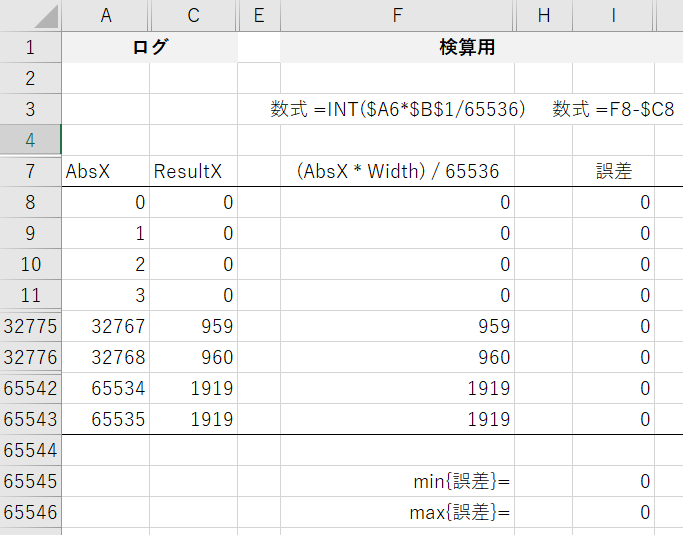(Since I have performed operations such as inserting rows, the cell reference of the formula is out of sync with the memo.)

## 3.3. Source code used in the experiment

Since the problem becomes complicated when affected by the coordinate system conversion by high resolution, we have made it compatible with high resolution with SetProcessDPIAware (Windows API).

#### SendInputMousePosTest.cs



using System;
using System.Drawing;
using System.IO;
using System.Runtime.InteropServices;
using System.Windows.Forms;

class MainForm : Form
{
readonly int sweepStep = 1; //X-coordinate base to send to SendInput

bool abortFlag;

//Termination x to send to SendInput,y coordinate. Set via command line arguments.
// (memo:The command line argument is received as an int, and it is long to prevent overflow in the for statement in the sweep.)
long _lastAbsX;
long _lastAbsY;

string _directionOption; // "d":Licking(Diagonal)(x,sweep y at the same time)other than that:Horizontal and vertical

MainForm(int lastAbsX, int lastAbsY, string directionOption)
{
abortFlag = false;
_directionOption = directionOption;
_lastAbsX = lastAbsX;
_lastAbsY = lastAbsY;

ClientSize = new System.Drawing.Size(300,100);
FormClosing += (s,e)=>{abortFlag=true;};
}

private static class NativeMethods
{
[DllImport("user32.dll", SetLastError = true)]
public extern static void SendInput(int nInputs, Input[] pInputs, int cbsize);

[DllImport("user32.dll", SetLastError = true)]
public extern static IntPtr GetMessageExtraInfo();

[DllImport( "user32.dll" )]
public static extern bool SetProcessDPIAware();
}

[StructLayout(LayoutKind.Sequential)]
private struct POINT
{
public int x;
public int y;
}

[StructLayout(LayoutKind.Sequential)]
struct MouseInput
{
public int X;
public int Y;
public int Data;
public int Flags;
public int Time;
public IntPtr ExtraInfo;
}

[StructLayout(LayoutKind.Sequential)]
struct KeyboardInput
{
public short VirtualKey;
public short ScanCode;
public int Flags;
public int Time;
public IntPtr ExtraInfo;
}

[StructLayout(LayoutKind.Sequential)]
struct HardwareInput
{
public int uMsg;
public short wParamL;
public short wParamH;
}

[StructLayout(LayoutKind.Sequential)]
struct Input
{
public int Type;
public InputUnion ui;
}

[StructLayout(LayoutKind.Explicit)]
struct InputUnion
{
[FieldOffset(0)]
public MouseInput Mouse;
[FieldOffset(0)]
public KeyboardInput Keyboard;
[FieldOffset(0)]
public HardwareInput Hardware;
}

private const int MOUSEEVENTF_MOVE        = 0x0001;
private const int MOUSEEVENTF_ABSOLUTE    = 0x8000;

void SendMouseMove()
{
BeginInvoke(
(MethodInvoker)delegate(){
checked{
var scrs = Screen.AllScreens;
for(int i=0;i<scrs.Length;i++) {
if(abortFlag){return;}

Console.Write("Size of screen[");
Console.Write(i);
Console.Write("]\t");
Console.Write(scrs[i].Bounds.Width);
Console.Write("\t");
Console.WriteLine(scrs[i].Bounds.Height);

Console.Write("Location of screen[");
Console.Write(i);
Console.Write("]\t");
Console.Write(scrs[i].Bounds.Left);
Console.Write("\t");
Console.WriteLine(scrs[i].Bounds.Top);
}

if ( _directionOption == "d" ) {
//upper left(0,0)From bottom right(_lastAbsX,_lastAbsY)Move diagonally to x,Move y at the same time to output the coordinates.
for ( long i = 0 ; i < _lastAbsX + sweepStep ; i += sweepStep ) {
if(abortFlag){return;}
long x = i;
if ( x > _lastAbsX ){x = _lastAbsX;}
long y = (x * _lastAbsY) / _lastAbsX;

SendInputMouseMoveAndDumpPoint((int)x, (int)y);
}
}
else {
//upper left(0,0)From(_lastAbsX,0)Move in a straight line to x,Output y.
for ( long i = 0 ; i < _lastAbsX + sweepStep ; i += sweepStep ) {
if(abortFlag){return;}
long x = i;
if (x > _lastAbsX) {x = _lastAbsX;}

SendInputMouseMoveAndDumpPoint((int)x, 0);
}

//upper left(0,0)From(0,_lastAbsY)Move in a straight line to x,Output y.
for ( long i = 0 ; i < _lastAbsY + sweepStep ; i += sweepStep ) {
if(abortFlag){return;}
long y = i;
if (y > _lastAbsY) {y = _lastAbsY;}

SendInputMouseMoveAndDumpPoint(0, (int)y);
}
}
}
MessageBox.Show("Completed.");
}
);
}

static void SendInputMouseMoveAndDumpPoint(int absX, int absY)
{
SendInputMouseMove(absX, absY);
DumpPoint(absX, absY);
}

static void DumpPoint(int orgAbsX, int orgAbsY)
{
Point p = Cursor.Position;
Console.Write(orgAbsX);
Console.Write("\t");
Console.Write(orgAbsY);
Console.Write("\t");
Console.Write(p.X);
Console.Write("\t");
Console.WriteLine(p.Y);
}

private static void SendInputMouseMove(int absX, int absY)
{
var mv = new Input();
mv.Type = 0; // MOUSE = 0
mv.ui.Mouse.X = absX;
mv.ui.Mouse.Y = absY;
mv.ui.Mouse.Data = 0;
mv.ui.Mouse.Flags = MOUSEEVENTF_ABSOLUTE | MOUSEEVENTF_MOVE;
mv.ui.Mouse.Time = 0;
mv.ui.Mouse.ExtraInfo = IntPtr.Zero;

Input[] inputs = new Input[]{mv};
NativeMethods.SendInput(inputs.Length, inputs, Marshal.SizeOf(inputs));
}

static void Main(string[] args)
{
NativeMethods.SetProcessDPIAware(); //To support high resolution scaling coordinates

int lastAbsX;
int lastAbsY;
string directionOption;

if ( args.Length==0 ) {
lastAbsX = 65535;
lastAbsY = 65535;
directionOption = "d";
}
else if ( args.Length==3 ) {
try{
lastAbsX = Convert.ToInt32(args);
lastAbsY = Convert.ToInt32(args);
}
catch(FormatException){
return;
}
catch(OverflowException){
return;
}
if(lastAbsX<0){return;}
if(lastAbsY<0){return;}

directionOption = args;
}
else {
return;
}

Application.Run(new MainForm(lastAbsX,lastAbsY,directionOption));
}
}


# 4. Then what should I do?

Relationships obtained in Chapter 3 Cursor.Position.X = (absX * Width) / 65536;
By performing the inverse calculation, the coordinate system of the mouse cursor is converted to the coordinate system of 0 to 65536.
I will go with the approach.

## 4.1. Floor function

The rounding process [^ 2] for integer division can be thought of as the floor function, as it is roughly the same as truncating the decimal point.

The graph of the floor function looks like this. (White circles are not included.)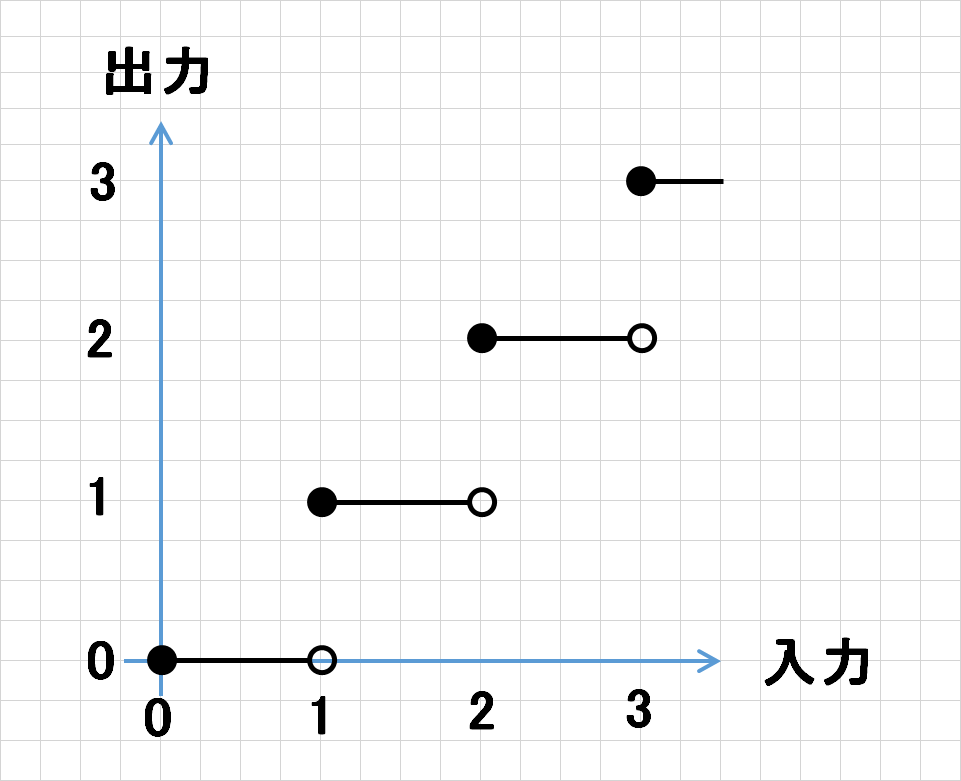## 4.2. Image of back calculation

Assuming that the Width and Height of the monitor are 65536 or less, the image of the reverse calculation when the actual example Width = 1920 is drawn as a graph as shown in the figure below.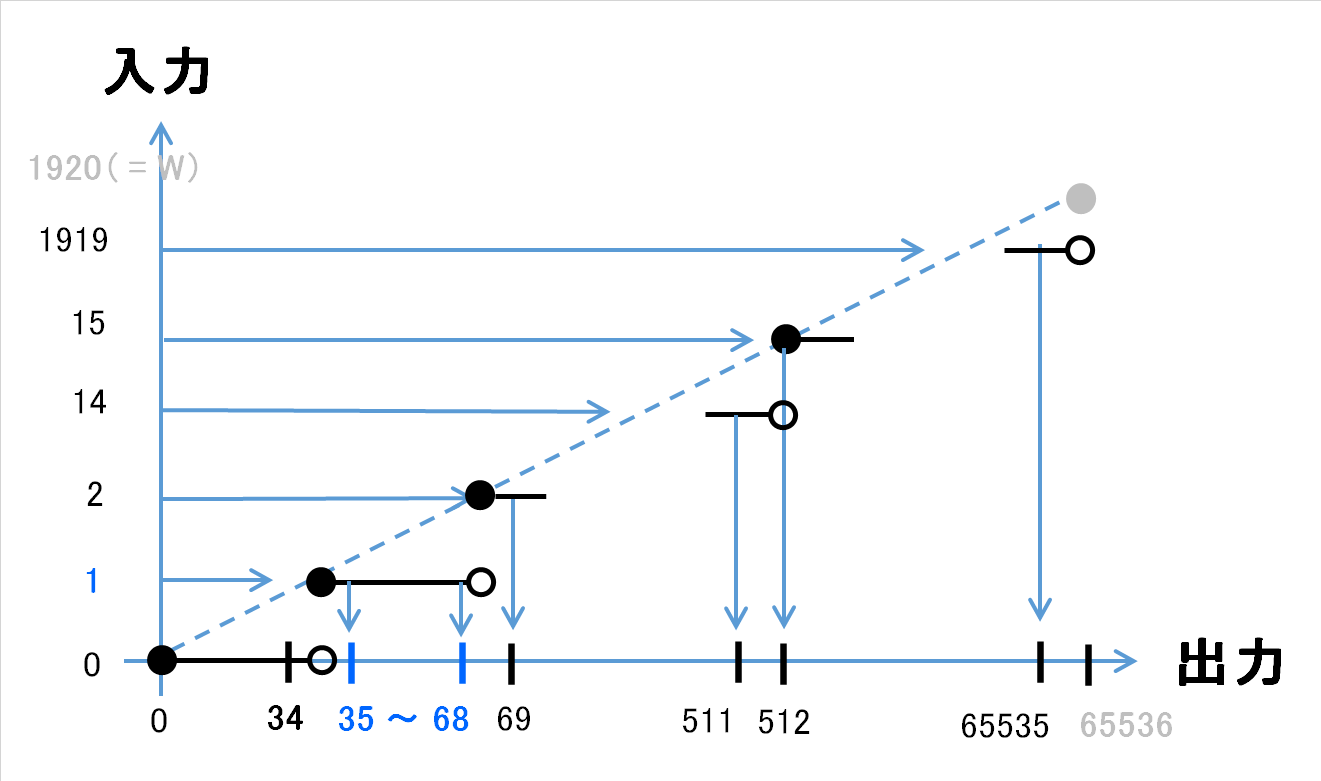If you want to move the cursor position x = 1, you need to set a value that falls within the range of 35 to 68 to absX of SendInput. [^ 3]
Therefore, it is necessary to construct an inverse calculation formula with a straight line that runs inside each line segment ● ー ○ on the graph. (vocabulary···)
It’s a little rough, but it’s the image below.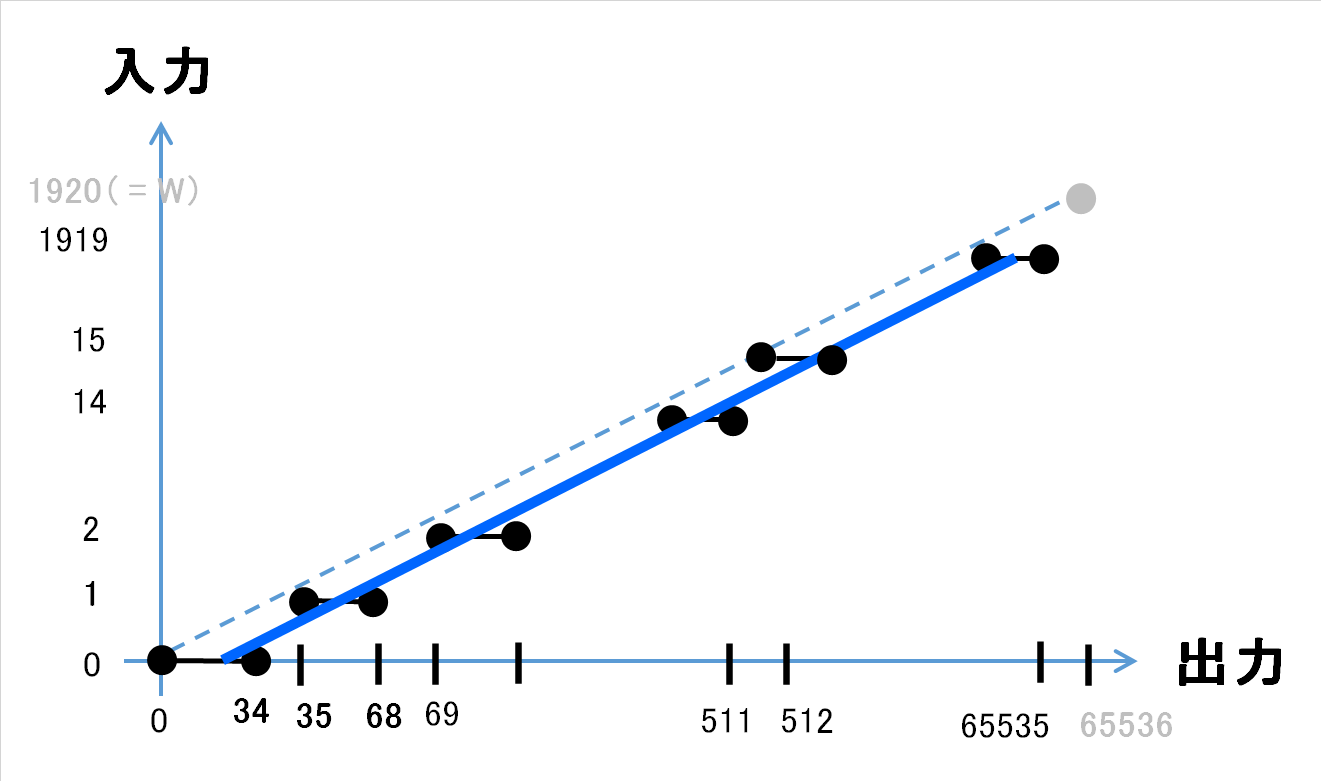[^ 3]: The official API description name is dx instead of absX. Since dx is associated with “difference” from the abbreviation image of differentiation and difference, absX is used in the article to make it easier to imagine absolute coordinates.

## 4.3. Inverse calculation formula

The story goes off, but if you rely on your intuition to come up with a formula, you can use the ceiling function.

absX = ceiling((Cursor.Position.X * 65536) ÷ Width)

I feel that it seems to be cool (it seems that the contents of Chapter 4.2. Can be satisfied) if it is calculated as (super violent). (Here, ÷ represents division by a real number.)
When I checked it with Excel, it seems that there is no error in the environment of Width = 1920, Height = 1080.

Image like this.

See the Pen Conversion graph of SendInput by kob58im (@kob58im) on CodePen.

## 4.4. Conclusion (code that could be converted accurately)

If the conversion formula in Chapter 4.3 is the source code (C #), it will be as follows.

The following "code that could be converted accurately" was finally issued in Chapter 4.3 with intuition , and whether or not the conversion mentioned in Chapter 4.2 was performed mathematically. Please use it as a reference as it has not been proved.

#### Code that could be converted accurately



absX = (x * 65536 + Screen.PrimaryScreen.Bounds.Width -1) / Screen.PrimaryScreen.Bounds.Width;
absY = (y * 65536 + Screen.PrimaryScreen.Bounds.Height-1) / Screen.PrimaryScreen.Bounds.Height;


Note: If x or y can take as large a value as 30000, cast it to a long type.

## 4.5. Try to prove

It’s a world of complete self-satisfaction, but I’m not calm unless I prove it, so I tried to prove it.

Generalize and put $N$ instead of 65536. Place the monitor width Width as $W$. Suppose $N \ geq W$.


p = \frac{N}{W}


And put it. Let $x$ be the x coordinate of the cursor.
At this time, the formula given as a proposal in Chapter 4.3 can be written as follows using the ceiling function $\ lceil x \ rceil$.


f(x) = \lceil x \cdot p \rceil



Also, if you write $g$ as the function that converts the coordinate system of SendInput to the x coordinate of the cursor, it can be expressed as follows using the floor function $\ lfloaor x \ rfloor$.


g(y) = \biggl\lfloor  y \cdot \frac{1}{p} \biggr\rfloor



In the following, $f (x)$ indicates that it takes the output value at the left end of each section of the graph at the bottom of Chapter 4.2.
In other words


g(f(x) -1) = x-1 \cdots\cdots ①



Prove that holds.
The above is drawn with the image of the graph in Chapter 4.2 as follows.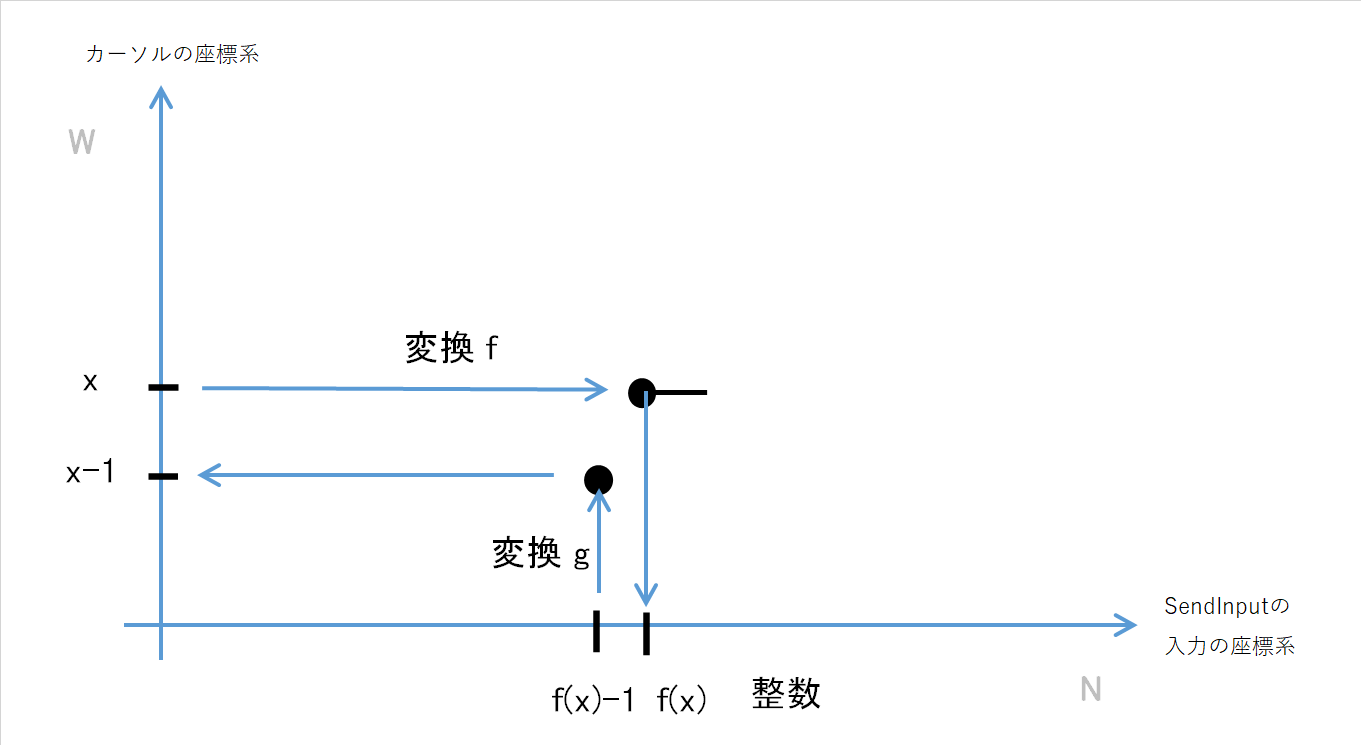If you expand $g$ in ①,


①   \Leftrightarrow\biggl\lfloor   (f(x) -1) \cdot \frac{1}{p} \biggr\rfloor  = x-1



If $\ lfloor y \ rfloor = C$, then $C \ leq y <C + 1$, so


①   \Leftrightarrow x-1 \leq   (f(x) -1) \cdot \frac{1}{p}   < x



Multiply all edges by $p$ and expand $f (x)$ to expand


① \Leftrightarrow (x-1)\cdot p \leq   \lceil x \cdot p \rceil -1   < x \cdot p



If you put $x \ cdot p$ as $z$ and add $1$ to all edges,


① \Leftrightarrow z-(p-1) \leq   \lceil z \rceil   < z + 1



Since $p -1 \ geq 0$, the above always holds. I was able to prove it with the above.

Tags:

Updated: